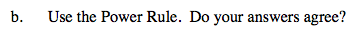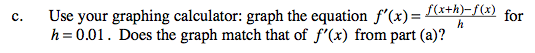### Home > CALC > Chapter 3 > Lesson 3.2.3 > Problem3-76

3-76.
1. Compare three different methods to find a derivative of f(x) =2x3x. Homework Help ✎

1. Use the definition of a derivative.

3. Use your graphing calculator: graph the equation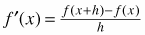for h = 0.01. Does the graph match that of f ′(x) from part (a)?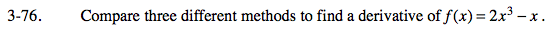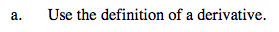Expand the numerator.

Combine like terms to simplify the numerator.

Factor out an h.

Simplify the fraction by 'cancelling out' the h.

Evaluate the limit as h→0.